# 题目

 1 2  输入: [3,2,1,5,6,4] 和 k = 2 输出: 5 

 1 2  输入: [3,2,3,1,2,4,5,5,6] 和 k = 4 输出: 4 

# 题解

0 1 2 3 4 5
3 2 1 5 6 4

0 1 2 3 4 5
2 1 5 6 4

0 1 2 3 4 5
1 2 ? 5 6 4

0 1 2 3 4 5
1 2 3 5 6 4

0 1 2 3 4 5
1 2 3 5 6 4

0 1 2 3 4 5
1 2 3 ? 6 4

0 1 2 3 4 5
1 2 3 4 6 ?

0 1 2 3 4 5
1 2 3 4 6

0 1 2 3 4 5
1 2 3 4 5 6

  1 2 3 4 5 6 7 8 9 10 11 12 13 14 15 16 17 18 19 20 21 22  void quick_sort(vector &nums, int l, int r) { if (l < r) { int i = l; int j = r; int tmp = nums[l]; while (i < j) { while (i < j && nums[j] > tmp) j--; if (i < j) nums[i++] = nums[j]; while (i < j && nums[i] < tmp) i++; if (i < j) nums[j--] = nums[i]; } nums[i] = tmp; quick_sort(nums, l, i - 1); quick_sort(nums, i + 1, r); } }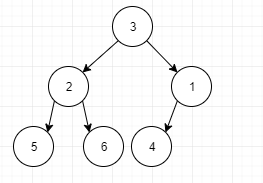1. 首先将数组堆化，即让数组满足堆的性质（父节点总是大于（小于）子节点）。
2. 将堆的顶点与末尾元素交换，之后对除末尾元素外剩下的元素继续重复该步骤，直到待堆化的元素数量为0.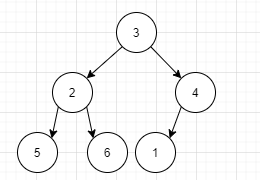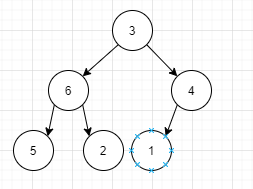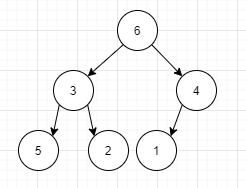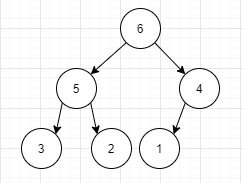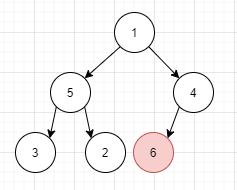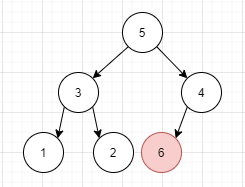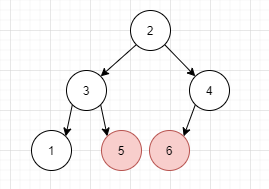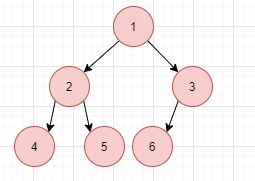1 2 3 4 5 6 7 8 9 10 11 12 13 14 15 16 17 18 19 20 21 22 23 24 25 26 27 28 29 30  void adjust(int *data, int i, int count) { int tmp = data[i]; for (int largest = i * 2 + 1; largest < count; largest = largest * 2 + 1) { if (largest != count - 1 && data[largest + 1] > data[largest]) largest++; if (data[largest] > tmp) { data[i] = data[largest]; i = largest; } else break; } data[i] = tmp; } void HeapSort(int *data, int count) { for (int i = count / 2 - 1; i >= 0; i--) { adjust(data, i, count); } for (int i = count - 1; i > 0; i--) { int tmp = data; data = data[i]; data[i] = tmp; adjust(data, 0, i); } } 

  1 2 3 4 5 6 7 8 9 10 11 12 13 14 15 16 17 18 19 20 21 22 23 24 25 26 27 28 29 30 31 32 33  void adjust(vector& data, int i, int count) { int tmp = data[i]; for (int largest = i * 2 + 1; largest < count; largest = largest * 2 + 1) { if (largest != count - 1 && data[largest + 1] > data[largest]) largest++; if (data[largest] > tmp) { data[i] = data[largest]; i = largest; } else break; } data[i] = tmp; } void HeapSort(vector& data, int count, int k) { for (int i = count / 2 - 1; i >= 0; i--) { adjust(data, i, count); } for (int i = count - 1, j = 1; i > 0; i--, j++) { int tmp = data; data = data[i]; data[i] = tmp; if (j == k) break; adjust(data, 0, i); } } 

# 参考文章

https://songlee24.github.io/2014/04/02/heap-sort-implementation/

https://zh.wikipedia.org/wiki/%E5%A0%86%E6%8E%92%E5%BA%8F

https://www.cnblogs.com/chengxiao/p/6129630.html